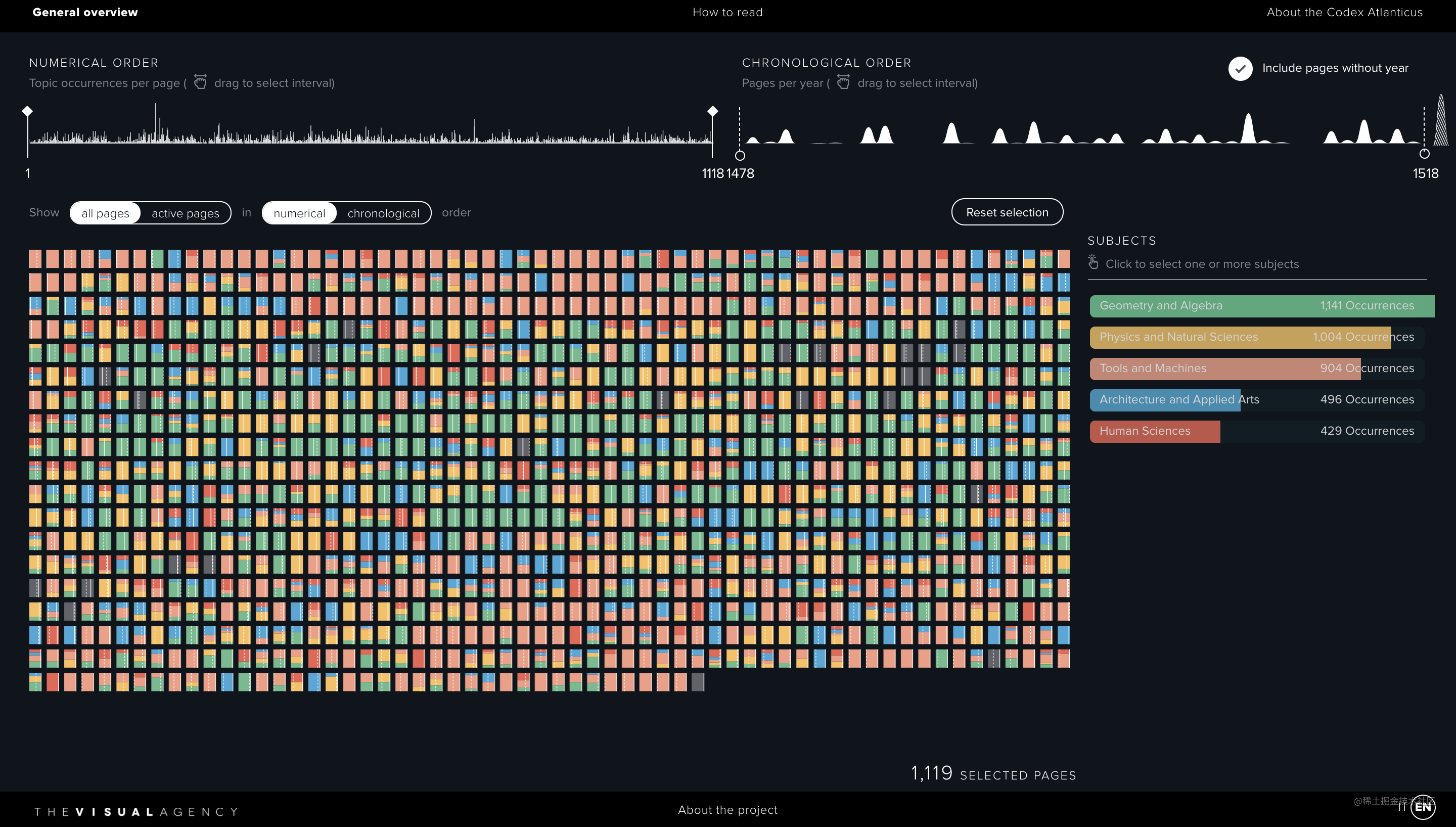# 手把手带你上手D3.js数据可视化系列（一）

## 前言## 正文

### 基本代码结构

``````<!DOCTYPE html>
<html lang="en">

<meta charset="UTF-8">
<meta http-equiv="X-UA-Compatible" content="IE=edge">
<meta name="viewport" content="width=device-width, initial-scale=1.0">
<title>D3.js 教程</title>
<style>
* {
margin: 0;
}

html,
body {
overflow: hidden;
}
</style>
<body>
<div id="chart"></div>
<!-- 可以下载到本地也可以引用线上版本 -->
<script src="./d3.js"></script>
<!-- <script src="https://cdn.bootcdn.net/ajax/libs/d3/5.9.7/d3.js"></script> -->
<script>
function drawChart() {
// code
}

drawChart()
</script>
</body>

### 添加 SVG 画布

`D3.js` 进行可视化，可以用矢量图的 SVG，也可以用标量图、像素的canvas，因为古柳 SVG 用的多些，这里就以此为例。

``````const width = window.innerWidth
const height = window.innerHeight

const svg = d3.select('#chart')
.append('svg')
.attr('width', width / 2)
.attr('height', height)
.style('background', '#FEF5E5')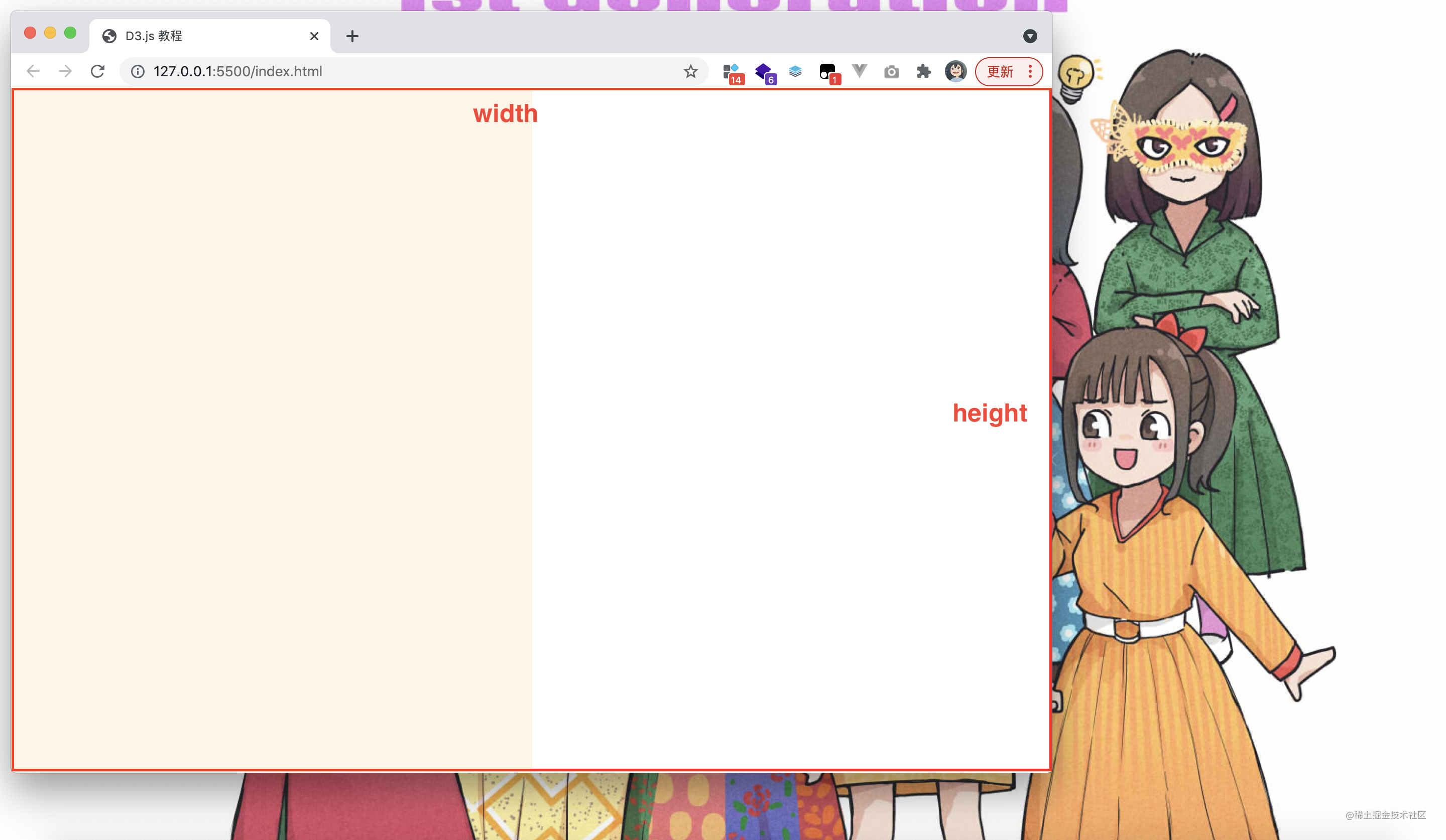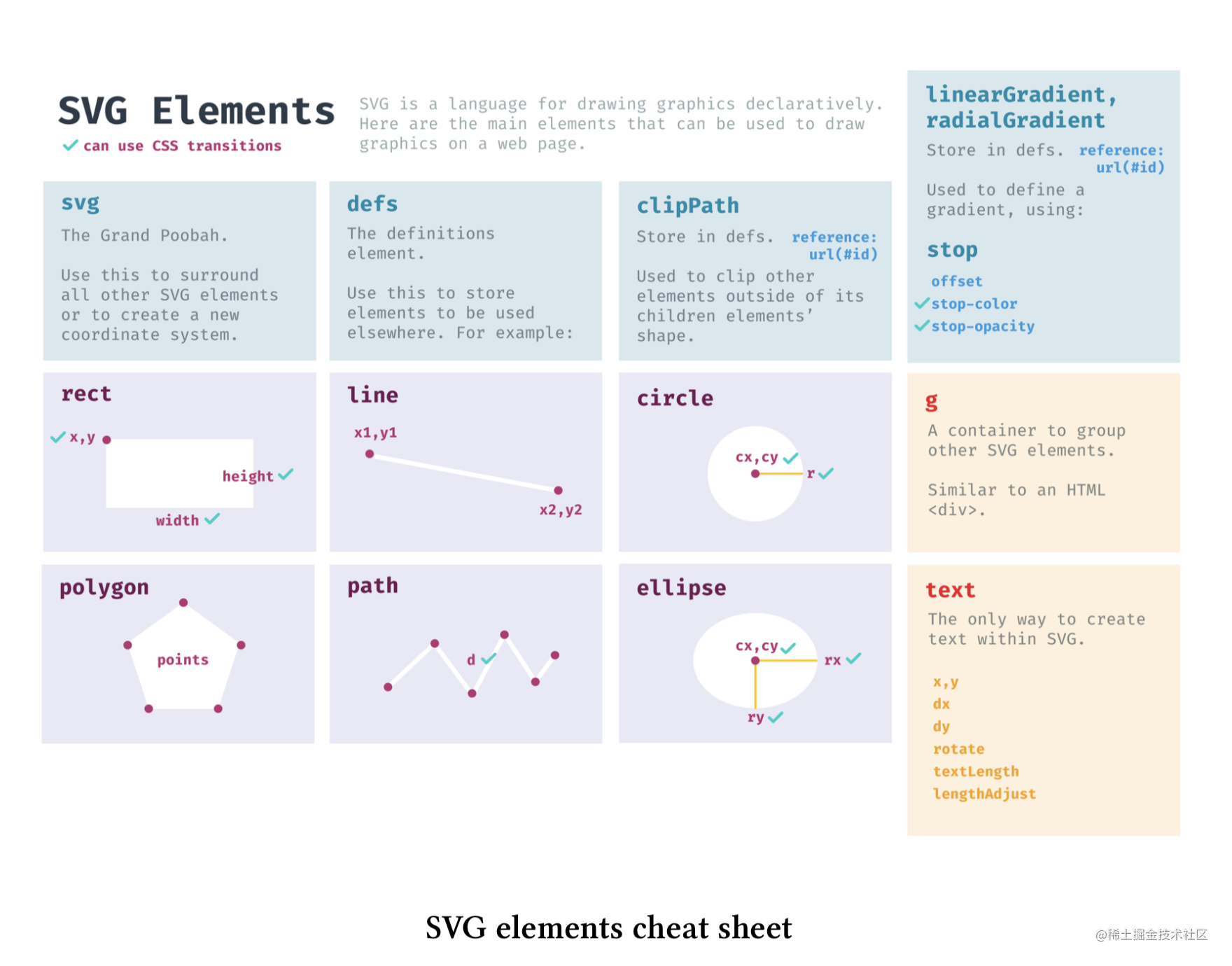``````svg.append('rect')
.attr('x', 30)
.attr('y', 50)
.attr('width', 50)
.attr('height', 100)
.attr('fill', '#00AEA6')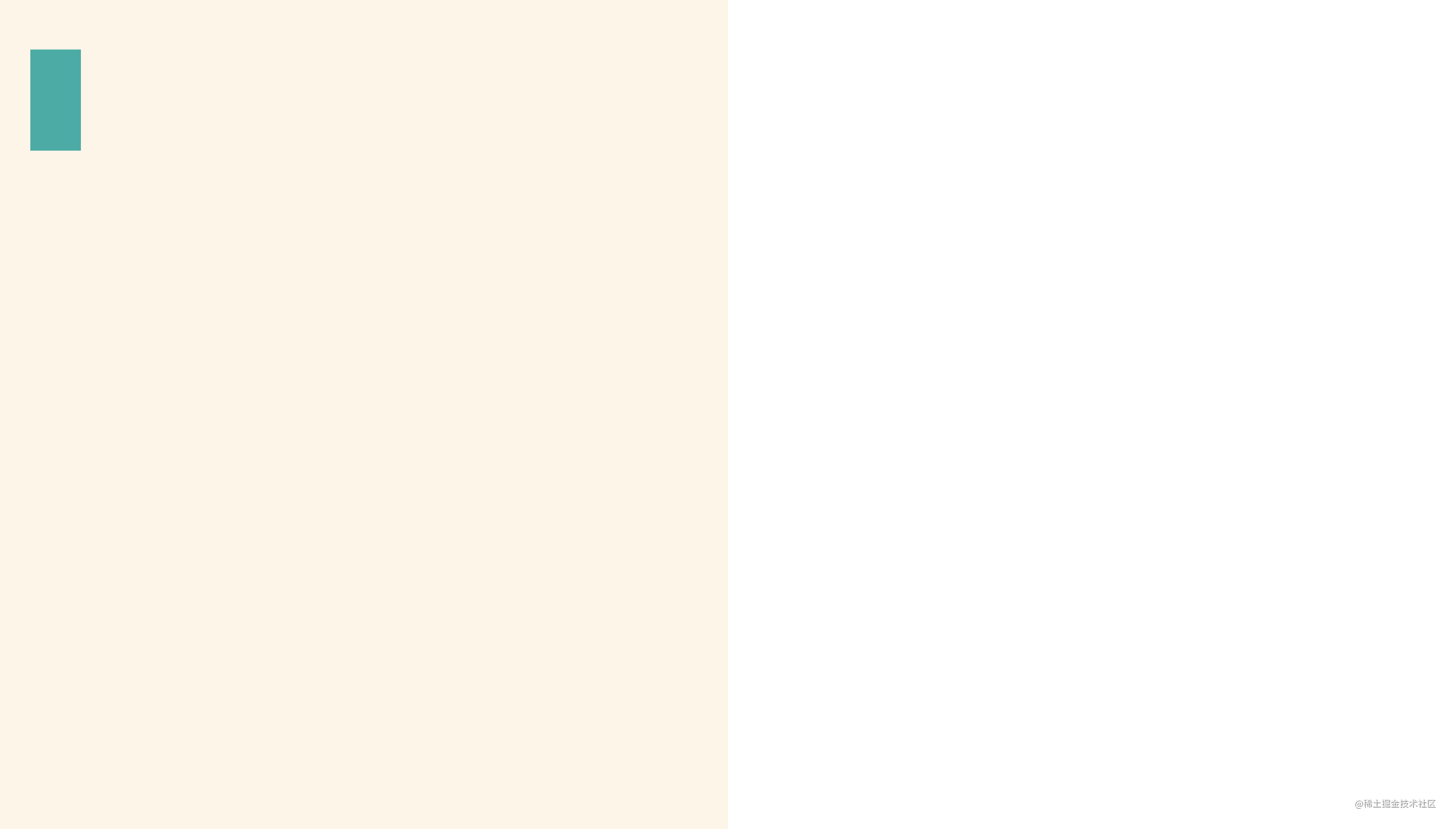``````<svg width="518.5" height="680" style="background: rgb(254, 245, 229);">
<rect x="30" y="50" width="50" height="100" fill="#00AEA6"></rect>
</svg>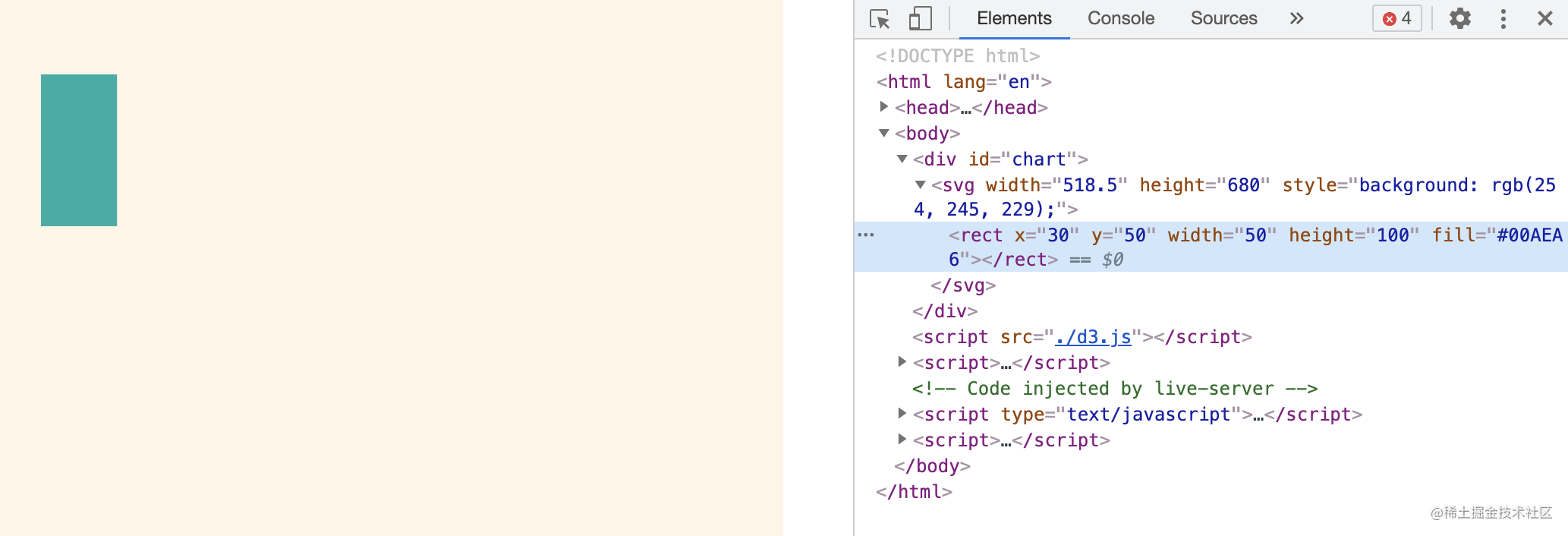``````svg.append('rect')
.attr('x', width / 2 - 25)
.attr('y', 50)
.attr('width', 50)
.attr('height', 100)
.attr('fill', '#EB5C36')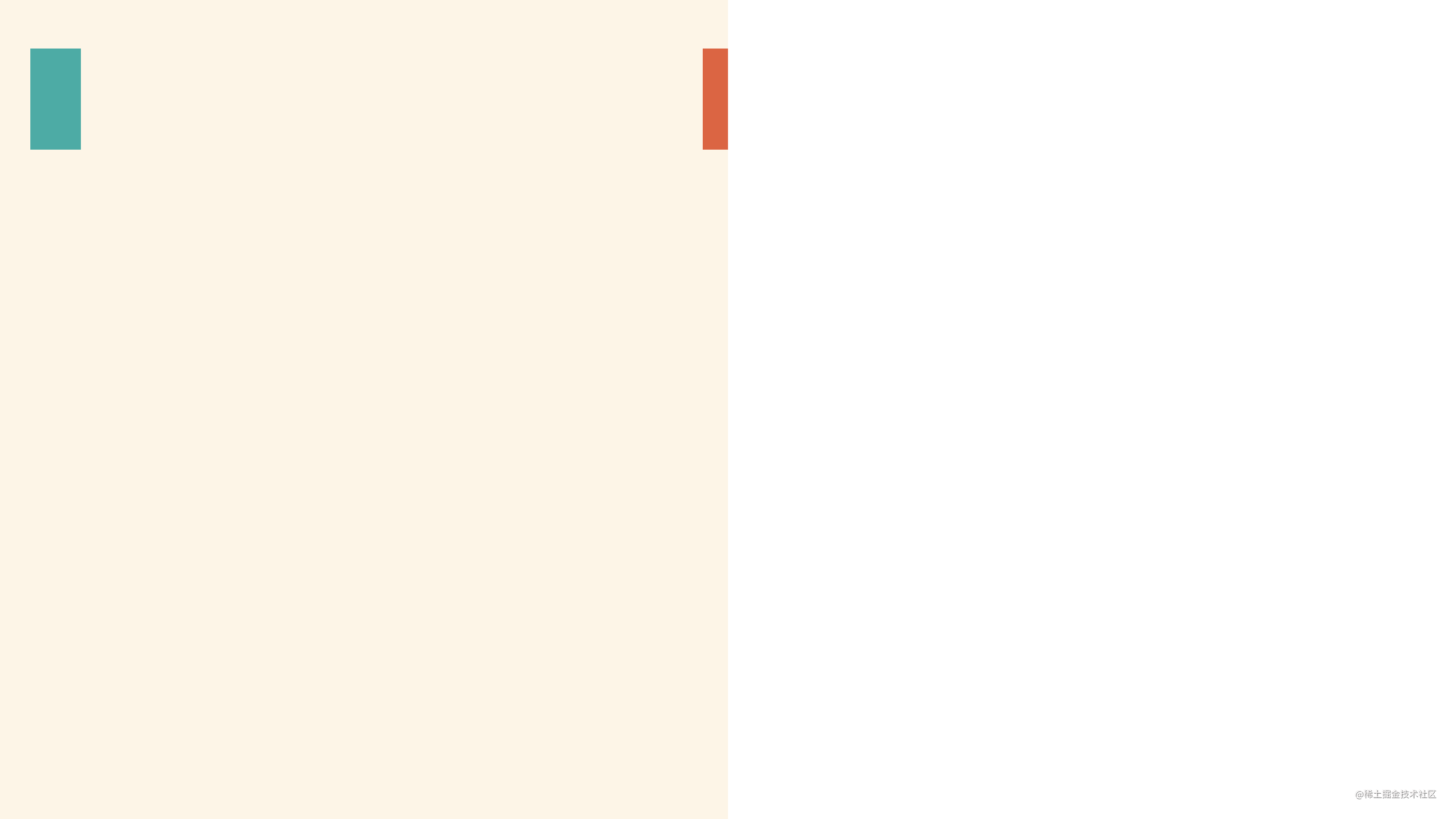### 构造简单数据

``````const dataset = d3.range(20)
console.log(dataset) // [0, 1, 2, ..., 19]

const colors = ['#00AEA6', '#DB0047', '#F28F00', '#EB5C36', '#242959', '#2965A7']### 遍历数据来添加元素

``````dataset.forEach(d => {
svg.append('rect')
.attr('x', 20 + d * 70)
.attr('y', 20)
.attr('width', 50)
.attr('height', 100)
.attr('fill', colors[d % colors.length])
})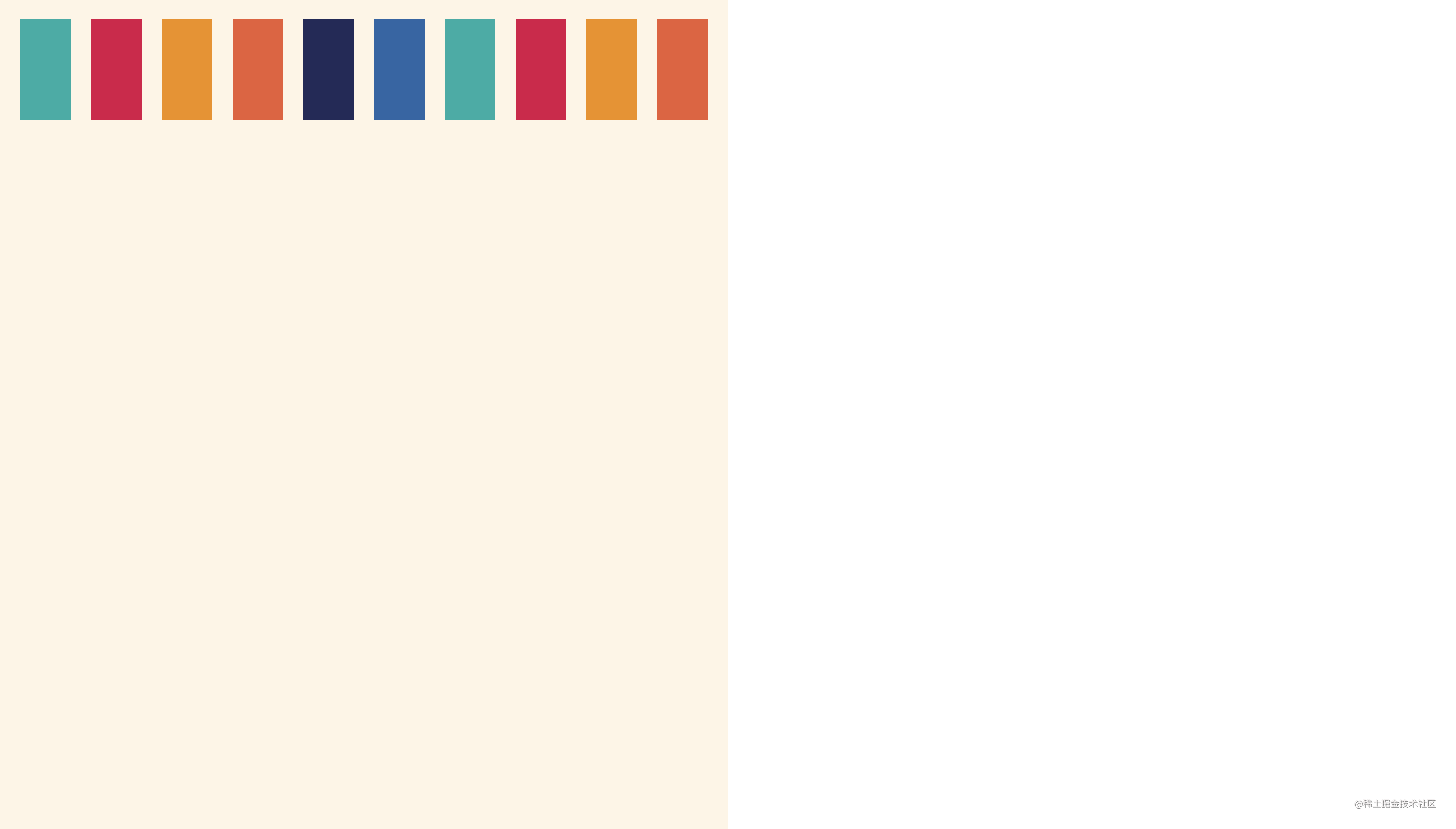``````0 % 6 => 0 => colors
1 % 6 => 1 => colors
2 % 6 => 2 => colors
...
5 % 6 => 5 => colors
6 % 6 => 0 => colors
7 % 6 => 1 => colors
...
19 % 6 => 1 => colors

### D3.js 基于数据添加元素的方式

``````const rects = svg.selectAll('rect')
.data(dataset)
.join('rect')
.attr('x', d => 20 + d * 70)
.attr('y', 20)
.attr('width', 50)
.attr('height', 100)
.attr('fill', d => colors[d % colors.length])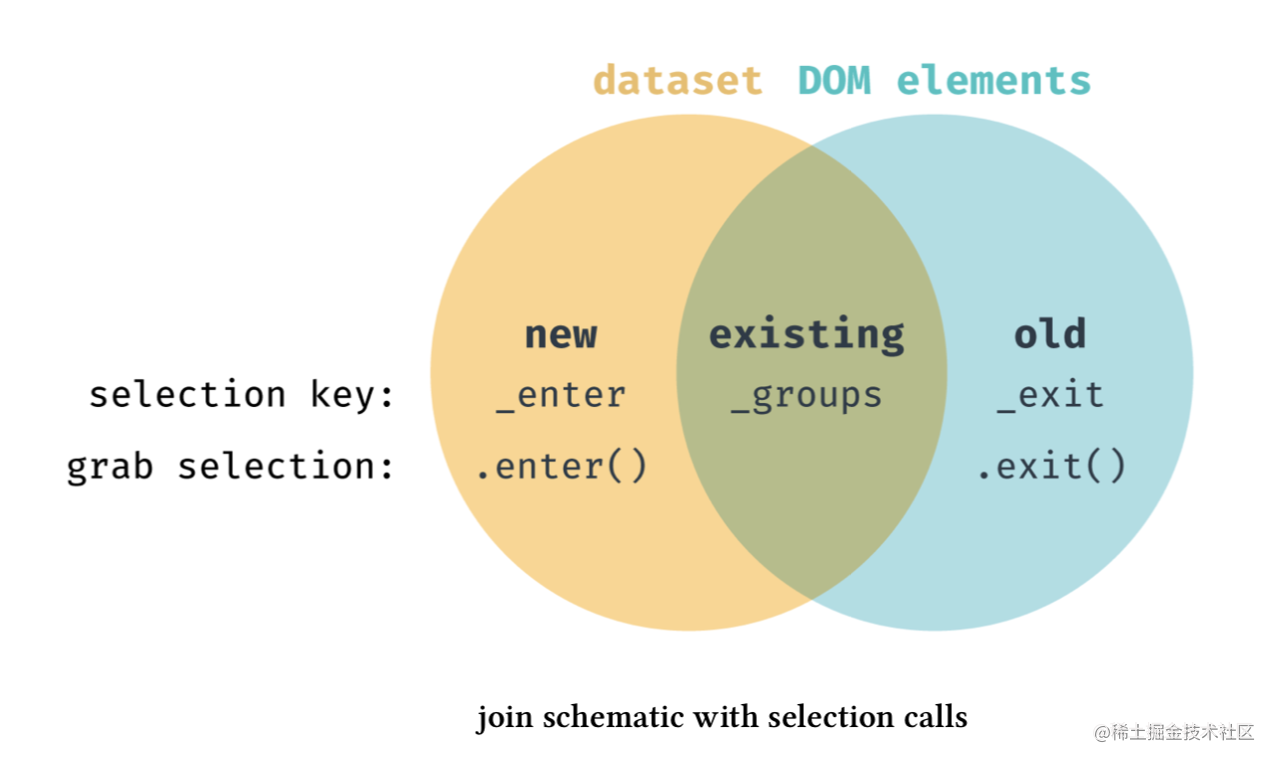``````const rects = svg.selectAll('rect')
.data(dataset)
.enter()
.append('rect')
.attr('x', function (d) {
return 20 + d * 70
})
.attr('y', 20)
.attr('width', 50)
.attr('height', 100)
.attr('fill', function (d) {
return colors[d % colors.length]
})

### 调整布局，换行显示

x 坐标的计算公式是 `20 + d * 70`，这里希望`每一行的最后一个矩形整体都在画布内，即 x 坐标加上矩形宽度要小于画布宽度`。由此可以计算出一行最多放多少个矩形，以 `col_num` 命名，注意这里第 n 个元素对于的 d 其实是 n-1，因为 d 是从0开始的，元素确实从第一个元素开始的。

``````// 公式
20 + (col_num - 1) * 70 + 50 <= witdh / 2

// 等同于
col_num <= witdh / 2 / 70

``````const col_num = parseInt(width / 2 / 70)
// const col_num = Math.floor(width / 2 / 70)
console.log(col_num)

``````const dataset = d3.range(50)

const rects = svg.selectAll('rect')
.data(dataset)
.join('rect')
.attr('x', d => 20 + d % col_num * 70)
.attr('y', d => 20 + Math.floor(d / col_num) * 120)
.attr('width', 50)
.attr('height', 100)
.attr('fill', d => colors[d % colors.length])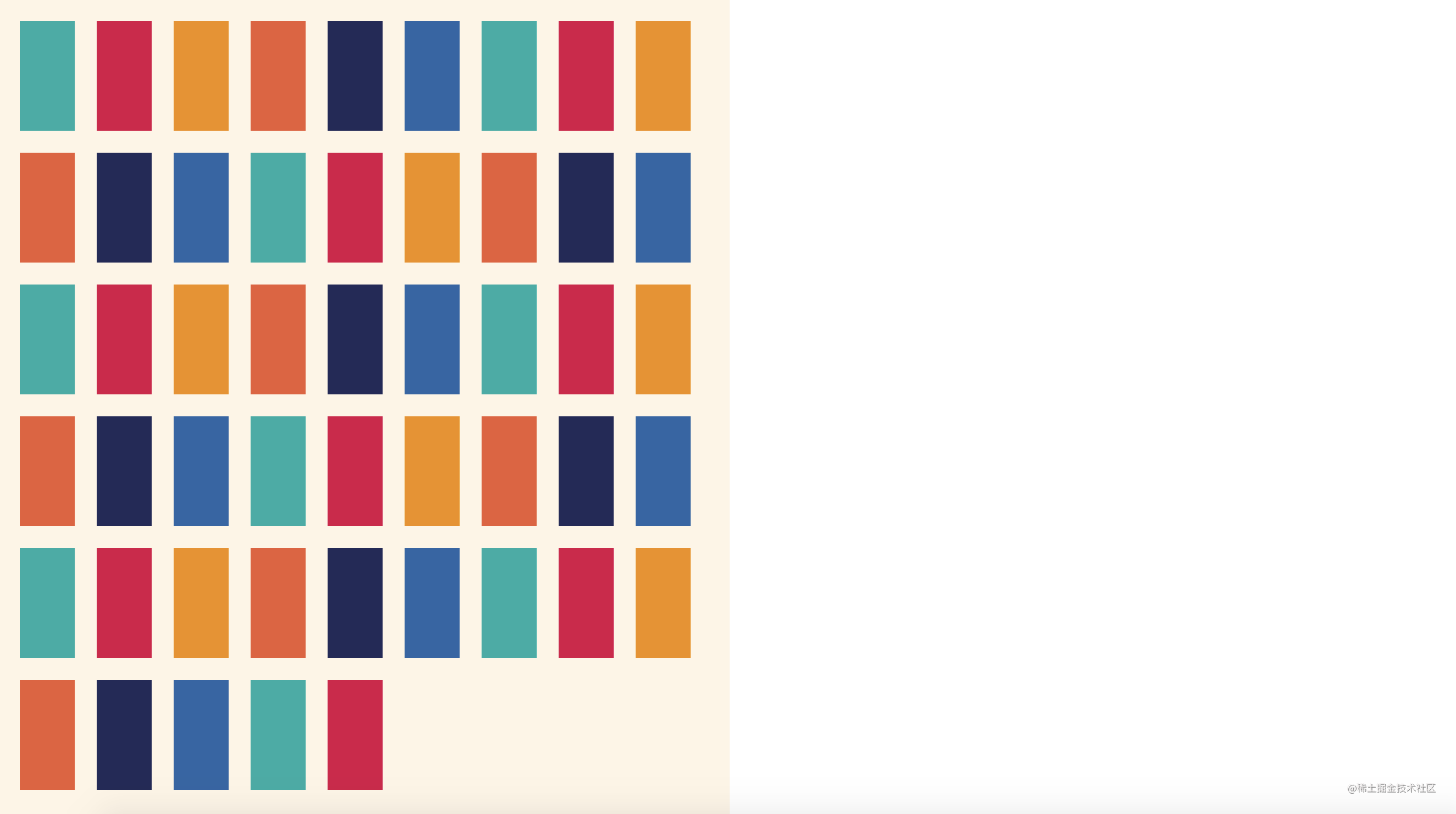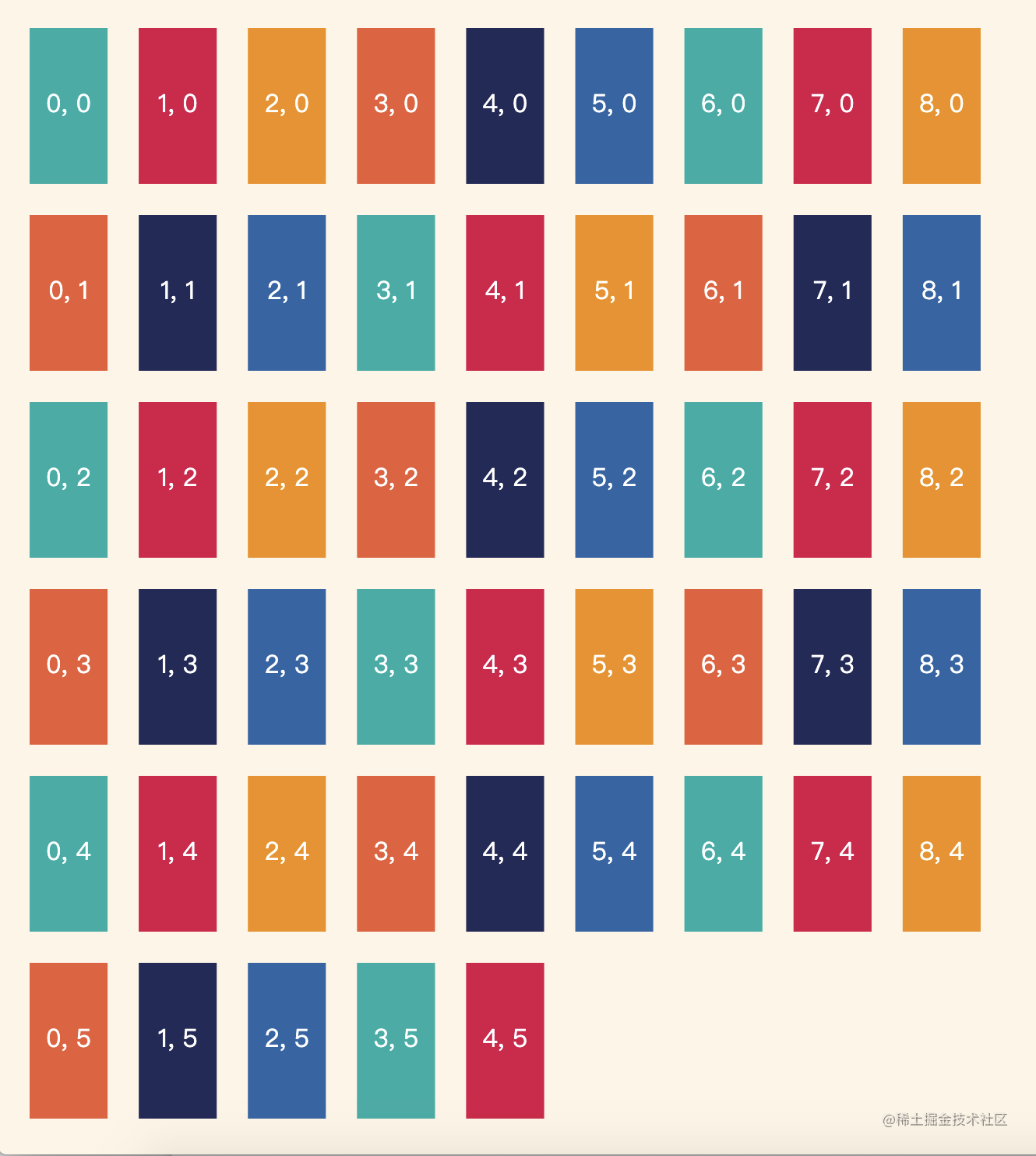``````const dataset = d3.range(100)

const rects = svg.selectAll('rect')
.data(dataset)
.join('rect')
.attr('x', d => 20 + d % col_num * 70)
.attr('y', d => 20 + Math.floor(d / col_num) * 120)
.attr('width', 50 / 2)
.attr('height', 100 / 2)
.attr('fill', d => colors[d % colors.length])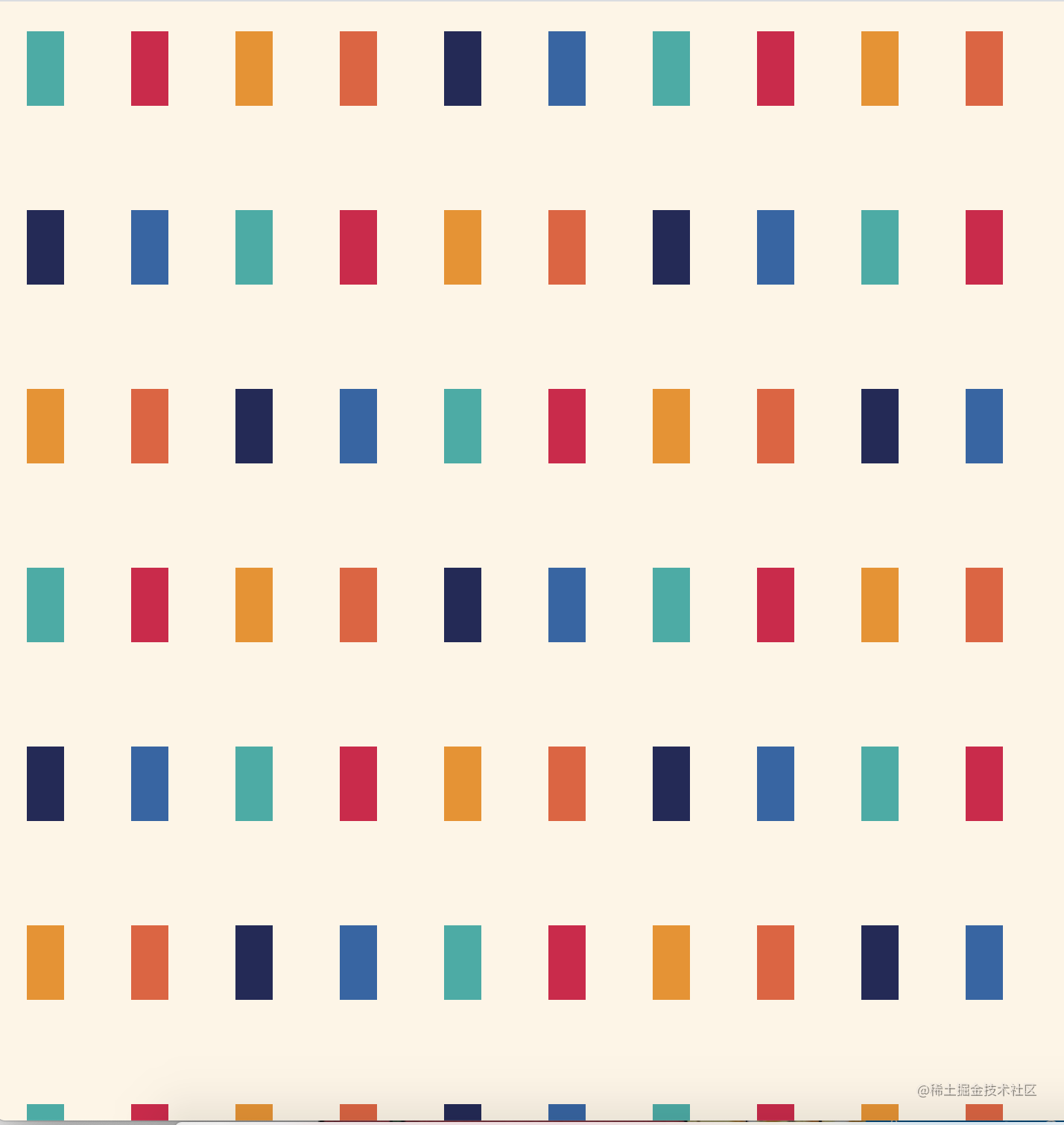`是否能基于数据大小和画布宽度来自动计算出每个rect的宽高和间距，然后自动布局呢？`